This post about solving radical equations is part of a series of posts to help you prepare for the Advanced Algebra and Functions part of the Accuplacer test.

## Question

Does the equation  have a real solution? If so, what is it?

## Solution

This kind of equation is called a radical equation, because it contains a radical — in this case, a square root.

Let’s try to solve this radical equation:

Subtract 5 from each side:

The square root of something equals something negative? Really? The definition of square root specifies that it means a positive number. But this square root is supposed to equal something negative.

No real solution.

You may be tempted to keep going from

and see what happens. OK, let’s try that. Follow the usual steps for solving a radical equation:

• Isolate the radical. We did that above when we added 10 to each side.
• Square both sides:

• Subtract 3 from each side:

• Divide by 4:

There’s a solution: . Now let’s see if it works. Substitute  back into the left-hand side of the original equation. You should get:

That simplifies to:

So what looked like the wrong way was indeed the wrong way, the solution  does not work, and the answer to the question is No, the equation  does not have a real solution.

This question is similar to question number 14 in the sample questions for the Accuplacer Advanced Algebra and Functions test.

## Is This Relation a Function?

This post about whether a relation is a function is part of a series of posts to help you prepare for the Advanced Algebra and Functions part of the Accuplacer test.

## Question

One or more of the graphs below represent 𝑦 as a function of 𝑥. Which one or ones?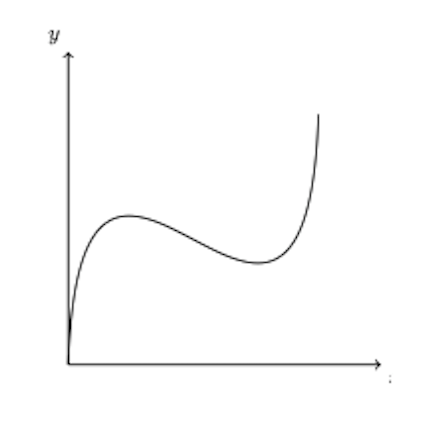AB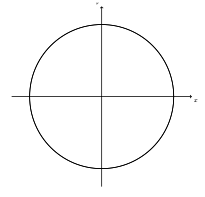CD

## Solution

Function/not function?

A function is a relation – that is, a correspondence between x and  y – for which every x-value relates to only one y-value. No vertical line will pass through more than one point on a function’s graph, but a vertical line may pass through more than one point on the graph of a relation that is not a function. Thus you can use the vertical line test on a relation to see whether it is a function.

Look at relation A. Imagine sliding a vertical line across it. At no point will the line intersect the relation more than once. This relation is a function.

Now look at relation B. The y-axis, which is a vertical line, intersects the relation in three places. Thus relation B fails the vertical line test and relation B is not a function.

Relation C: Again, there are places where you could draw a vertical line and cross the relation more than once. For example, the y-axis crosses the relation twice. This relation is not a function.

Relation D: There is only one x-value where it looks like a vertical line could cross this relation twice: the x-value at the right-hand end of the lower piece, which is the x-value at left-hand end of the upper piece. Are there two y-values at this x-value? The lower piece, in which the circle is filled in, does take this x-value. But the upper piece, in which the circle is not filled in, does not take the value. Thus there is no x-value that both pieces take, and there is no x-value for which there is more than one value of  This relation is a function.

𝑨𝒏𝒔𝒘𝒆𝒓: A and D

## Multiplying a Binomial by a Trinomial

This post about multiplying a binomial by a trinomial is part of a series of posts to help you prepare for the Advanced Algebra and Functions part of the Accuplacer test.

Multiply:

## Solution

The first expression — the contents of the first set of parentheses — is called a binomial because it has two terms. The second expression — the contents of the second set of parentheses — is called a trinomial because it has three terms. To multiply a binomial  by a trinomial, multiply each term in the binomial by each term in the trinomial.

You need a way to organize this. Try this: Multiply x, the first term in the binomial, by each term in the trinomial. Then multiply -2, the second term in the binomial, by each term in the trinomial. Then add all those results together.

• Multiply x, the first term in the binomial, by each term in the trinomial.

• Multiply -2, the second term in the binomial, by each term in the trinomial.

• Add the results, adding like terms to like terms. If you stack the expressions vertically, that will help you keep like terms lined up:

This question is similar to question number 4 in the sample questions for the Accuplacer Advanced Algebra and Functions test.

## End Behavior, Degree, and Leading Coefficient

This post about end behavior, degree, and leading coefficient of a polynomial function is part of a series of posts to help you prepare for the Advanced Algebra and Functions part of the Accuplacer test.

## Question

Consider the function

where a and c are integers and are constants and c is positive. The the graph y = g(x) goes toward negative infinity at both negative and positive extremes of x. Is positive or negative? Is c even or odd?

## Solution

Let’s deal with c first.

### Sidebar: even and odd polynomial functions

The end behavior of a polynomial function with an even degree is in the same direction — either toward negative infinity or toward positive infinity — for both extremes of x. Think, for example, of  . It goes up toward positive infinity as the absolute value of x increases, whether positive or negative. So do , , etc.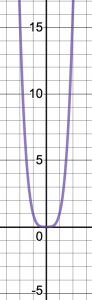If you multiply any of those expressions by a leading coefficient of -1, or any negative number, then end behavior goes to negative infinity for both extremely negative and extremely positive values of x.

On the other hand, the end behavior of a polynomial with an odd degree is in opposite directions for extremely negative and extremely positive values of x. Think of , , etc.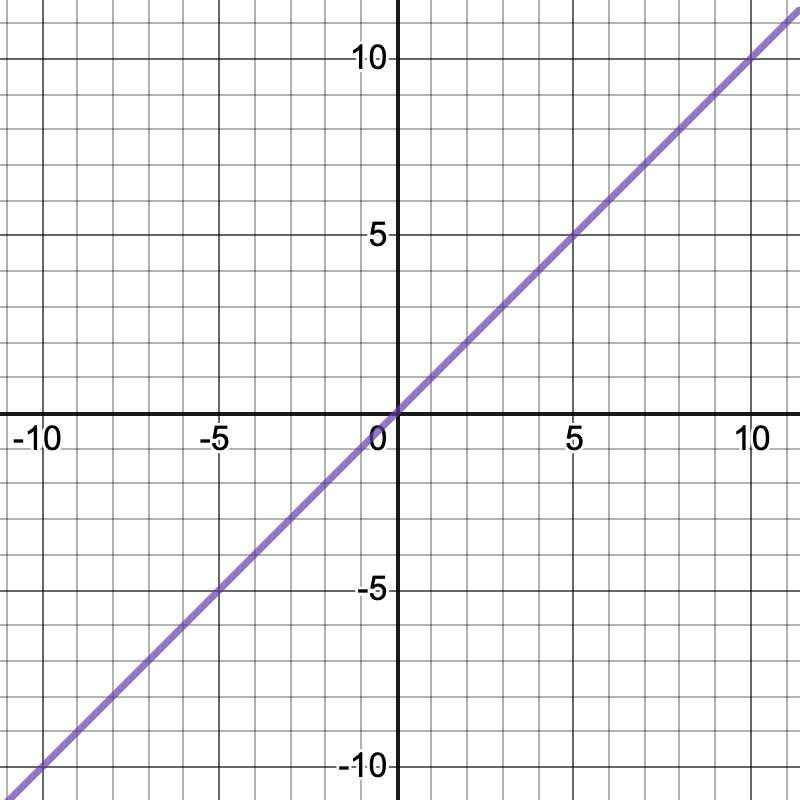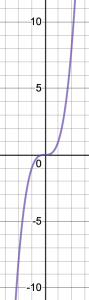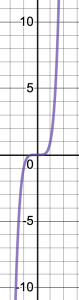So far, I’ve been talking about a monomial —  or  or y equals x to some other power. What happens in the more general case, when you get a polynomial with several terms, for example ?When a polynomial contains terms of lower degree than the leading term, you get some noise, but end behavior is still determined by the highest-degree term. Note that as x gets very negative or very positive, the graph of  starts to look more like the graph of .

### Back to the Question

The function in question, , has degree c + 1: The c comes from the power c on the term x + 4, and the +1 comes from the implied power of 1 on the term x – 3, for a total power of x  equal to c + 1. Thus c + 1 is the degree of function g. And you’re told that this function’s end behavior is to approach negative infinity at both extremes of x. As shown above, a polynomial that goes in the same direction — either up or down — for both extremely positive and extremely negatives values of x has an even degree. That means c+1 is even, so c is odd.

What about a? As shown above, a polynomial with even degree, like this one, that goes down at both extremes of x starts with a negative number. So a is negative.

This question is similar to question number 17 in the sample questions for the Accuplacer Advanced Algebra and Functions test.